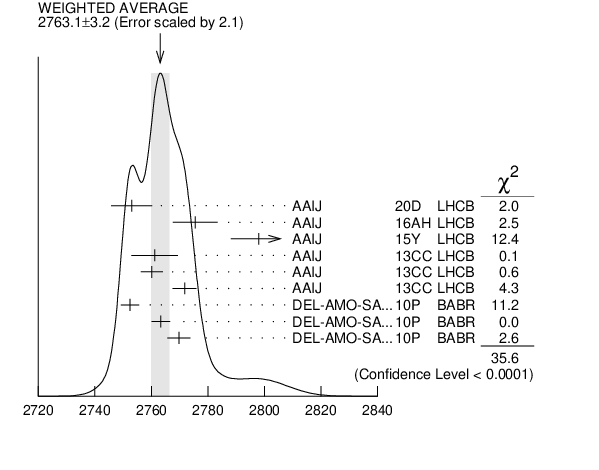# ${{\boldsymbol D}_{{3}}^{*}{(2750)}}$ MASS INSPIRE search

VALUE (MeV) EVTS DOCUMENT ID TECN CHG  COMMENT
$\bf{ 2763.5 \pm3.4}$ OUR AVERAGE  Error includes scale factor of 2.2.
$2775.5$ $\pm4.5$ $\pm6.5$ 28k 1
 2016 AH
LHCB ${{\mathit B}^{-}}$ $\rightarrow$ ${{\mathit D}^{+}}{{\mathit \pi}^{-}}{{\mathit \pi}^{-}}$
$2798$ $\pm7$ $\pm7$ 2
 2015 Y
LHCB ${{\mathit B}^{0}}$ $\rightarrow$ ${{\overline{\mathit D}}^{0}}{{\mathit \pi}^{+}}{{\mathit \pi}^{-}}$
$2761.1$ $\pm5.1$ $\pm6.5$ 14k
 2013 CC
LHCB 0 ${{\mathit p}}$ ${{\mathit p}}$ $\rightarrow$ ${{\mathit D}^{*+}}{{\mathit \pi}^{-}}{{\mathit X}}$
$2760.1$ $\pm1.1$ $\pm3.7$ 56k
 2013 CC
LHCB 0 ${{\mathit p}}$ ${{\mathit p}}$ $\rightarrow$ ${{\mathit D}^{+}}{{\mathit \pi}^{-}}{{\mathit X}}$
$2771.7$ $\pm1.7$ $\pm3.8$ 20k
 2013 CC
LHCB + ${{\mathit p}}$ ${{\mathit p}}$ $\rightarrow$ ${{\mathit D}^{0}}{{\mathit \pi}^{+}}{{\mathit X}}$
$2752.4$ $\pm1.7$ $\pm2.7$ 23.5k 3
 2010 P
BABR 0 ${{\mathit e}^{+}}$ ${{\mathit e}^{-}}$ $\rightarrow$ ${{\mathit D}^{*+}}{{\mathit \pi}^{-}}{{\mathit X}}$
$2763.3$ $\pm2.3$ $\pm2.3$ 11.3k 3
 2010 P
BABR 0 ${{\mathit e}^{+}}$ ${{\mathit e}^{-}}$ $\rightarrow$ ${{\mathit D}^{+}}{{\mathit \pi}^{-}}{{\mathit X}}$
$2769.7$ $\pm3.8$ $\pm1.5$ 5.7k 4, 3
 2010 P
BABR + ${{\mathit e}^{+}}$ ${{\mathit e}^{-}}$ $\rightarrow$ ${{\mathit D}^{0}}{{\mathit \pi}^{+}}{{\mathit X}}$
• • • We do not use the following data for averages, fits, limits, etc. • • •
$2802$ $\pm11$ $\pm10$ 5
 2015 Y
LHCB ${{\mathit B}^{0}}$ $\rightarrow$ ${{\overline{\mathit D}}^{0}}{{\mathit \pi}^{+}}{{\mathit \pi}^{-}}$
1  From the amplitude analysis in the model describing the ${{\mathit D}^{+}}{{\mathit \pi}^{-}}$ wave together with virtual contributions from the ${{\mathit D}^{*}{(2007)}^{0}}$ and ${{\mathit B}^{*0}}$ states, and components corresponding to the ${{\mathit D}_{{2}}^{*}{(2460)}^{0}}$, ${{\mathit D}_{{1}}^{*}{(2680)}^{0}}$, ${{\mathit D}_{{3}}^{*}{(2760)}^{0}}$, and ${{\mathit D}_{{2}}^{*}{(3000)}^{0}}$ resonances.
2  Modeling the ${{\mathit \pi}^{+}}{{\mathit \pi}^{-}}$ ${\mathit S}{\mathrm -wave}$ with the Isobar formalism.
3  The states observed in the ${{\mathit D}^{*}}{{\mathit \pi}}$ and ${{\mathit D}}{{\mathit \pi}}$ final states are not necessarily the same.
4  At a fixed width of 60.9 MeV.
5  Modeling the ${{\mathit \pi}^{+}}{{\mathit \pi}^{-}}$ ${\mathit S}{\mathrm -wave}$ with the K-matrix formalism.${{\mathit D}_{{3}}^{*}{(2750)}}$ MASS (MeV)
References:
 AAIJ 2016AH
PR D94 072001 Amplitude analysis of ${{\mathit B}^{-}}$ $\rightarrow$ ${{\mathit D}^{+}}{{\mathit \pi}^{-}}{{\mathit \pi}^{-}}$ Decays
 AAIJ 2015Y
PR D92 032002 Dalitz Plot Analysis of ${{\mathit B}^{0}}$ $\rightarrow$ ${{\overline{\mathit D}}^{0}}{{\mathit \pi}^{+}}{{\mathit \pi}^{-}}$ Decays
 AAIJ 2013CC
JHEP 1309 145 Study of ${{\mathit D}_{{J}}}$ Meson Decays to ${{\mathit D}^{+}}{{\mathit \pi}^{-}}$, ${{\mathit D}^{0}}{{\mathit \pi}^{+}}$ and ${{\mathit D}^{*+}}{{\mathit \pi}^{-}}$ Final States in ${{\mathit p}}{{\mathit p}}$ Collisions
 DEL-AMO-SANCHEZ 2010P
PR D82 111101 Observation of New Resonances Decaying to ${{\mathit D}}{{\mathit \pi}}$ and ${{\mathit D}^{*}}{{\mathit \pi}}$ in Inclusive ${{\mathit e}^{+}}{{\mathit e}^{-}}$ Collisions near $\sqrt {s }$ = 10.58 GeV